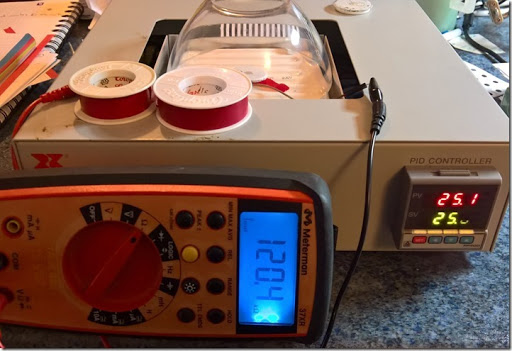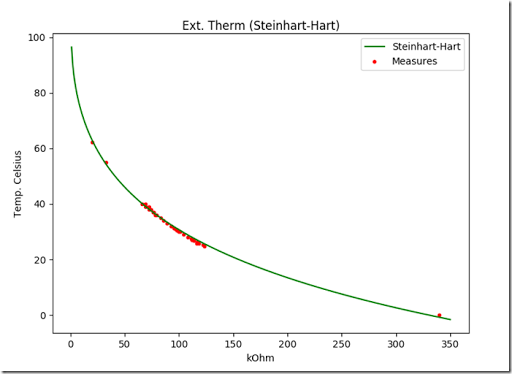## Wednesday, November 30, 2016

### Freestyle Libre external thermistor data dump

I’ve received a couple of questions about the Libre thermistor temperature curves in my previous blog post. One of the reason I did not post actual data is that I wasn’t 100% sure of their absolute validity (in relative terms, they were enough for my purpose). The data I used last year was derived through a polynomial fit, not the ideal option, when there is a better approximation formula that is commonly used for NTC thermistors.
That is why, today, I decided to double check the data and provide it.
Usual caveats
• thermistor have tolerances, 5%, 10%, generating errors.
• my multimeter probably suffers for a certain error.
• my contraption may introduce additional resistance.
• my heating bed’s thermistor also has its own error.
• the thermistor is extremely reactive: breathing on it changes its resistance, testing it in an open environment or under some insulation changes the values.
• I have seen thermistors not behaving as expected.
As usual, take your own measures.
Here is the test environment. The glass bowl serves as a temperature stabilizer. I am patching on an old Libre sensor.I ran a few measures at 55°C, 35°C, 0°C (separately), averaged them and solved the 3 equation system
1/T1 = a +b(log(R1))+c(log(R1))^3
1/T2 = a +b(log(R2))+c(log(R2))^3
1/T3 = a +b(log(R3))+c(log(R3))^3
to derive the Steinhart-Hart coefficients (working in kOhms rather than Ohms.
The Wolfram Alpha query for that system is
1/328=x+y*(ln(31.5))+z*(ln(31.5))^3, 1/308 = x +y*(ln(83))+z*(ln(83))^3, 1/273= x +y*(ln(330))+z*(ln(330))^3
It matches closely the solution I got in numpy. Here’s the plotted “theoretical“ curve versus the separately observed data. Please note that this can’t be directly converted in the Libre bits and bytes and is not necessarily exactly what the Libre sees.
The thermistor seems to be a 120k NTC thermistor and not a 100k thermistor. That was either a quick wrong assumption back in 2015, or a thermistor variation (I don’t remember and don’t have that thermistor for a retest anyway).

Edit 30/11 – after additional measures with a third thermistor, taking the gradient over my bed in consideration and testing older thermistors, the old assumption of a 100k thermistor could very well be the correct one, in that case, the curve below simply shifts to the left. It is correct in relative, but not absolute termsAnd here is is the python code containing the experimental data. Again, if you are interested, by all means, take your own measures (and eventually let me know). I have observed slightly different values, possibly a 100k NTC and, at least in one case, drastically different values. It is hard for me to know if the thermistor was damaged of if Abbott uses two different types. And yes, I am aware that the decimals in the data dump are probably pointless, but I just noted the values as the rig stabilized.
```import matplotlib.pyplot as plt
import numpy as np
import math as m

# 0C
K = 273

# takes the coefficients, measured resistance, returns temp in celsius (note kOhms)
def SteinhartHart(a, b, c, r):
invT = a + b * m.log(r) + c * (m.pow(m.log(r), 3))
TK = 1 / invT
T = TK - K
return T

# thermistor measures
Observed = [(40, 69), (40, 66), (39, 72), (39, 69), (38, 74), (38, 72), (37, 76), (37, 76.5), (36, 79),
(36, 78.34), (35, 83), (34, 86), (33, 89), (32, 93), (32, 93), (31, 96.8), (31.3, 95), (30.5, 98),
(30.5, 99), (29.9, 101), (27.6, 111), (27, 114), (25.8, 116.6), (25.2, 122.2), (25.9, 117.4),
(30, 100), (29, 104), (28, 108), (27, 112), (26.7, 114), (26.2, 116.8), (25.7, 118.4), (24.8, 123.3),
(55, 32.9), (62.2, 20), (0.1, 340), ]

fig = plt.figure()
ax.set_title('Ext. Therm (Steinhart-Hart)')
ax.set_xlabel('kOhm')
ax.set_ylabel('Temp. Celsius')

# plot observed data
v, t = zip(*Observed)
line2 = ax.scatter(t, v, c='r', marker='.', label="Measures")

# steinhart - hart, linalg code omitted, double checked with wolfram alpha kOhms
a = 0.00270712
b = 0.0000629848
c = 0.00000302854

# generates Steinhart-Hart Curve
x = np.linspace(1, 350, 350)
s = []
for i in x:
s.append((SteinhartHart(a, b, c, i)))
line = ax.plot(x, s, c='g', label="Steinhart-Hart")
plt.legend()
plt.show()```
` `

1.Hi Pierre, where can I found the thermistor value in libre sensor memory? if it's there

2.Have you noticed that the greenish SMD component that ist soldered in series to the other 999 Ohm resistor is a thermistor as well? So there are actually 3 possible temperature measuring sites on the libre PCB.

3.Thanks Rebecca! and sorry I missed your comment (went into spam for some reason). I had not noticed. I'll try to figure out how it fits in the big picture.

1.Hi Pierre,
This also means that the pin configuration of the rf430tal152h is different from the rf430frl152h (as far as I understand): the ADC Pins are located at Pin 13, Pin 18 (resistive bias) and Pin 19 (also resistive bias). SVSS seems to be on Pin 11 (at least all sensors are somehow connected to this Pin). There is no reference resistor for a self calibrating temperature measurement like in the reference design. So there must be calibration data for this, stored in FRAM.
From the information I could gather from websites (I could not get hands on a working sensor yet, as I am not diabetic at the moment. I could only study the electrical paths and components on a sensor with damaged antenna), only two temperature values are saved on the libre. So there might not even be a TI thermistor.

Thanks for sharing the results of your investigations!

4.We can probably continue this conversation privately if you want, just mail me. The FRAM is essentially known, except for two bytes. There is also the SRAM though.

As far as the designs are concerned, they could run with one, two or three thermistors (the third one at the measure site for example,as with designs that pulse a platinum wire and use it as rtd).

The skin thermistor is clearly there, known and behaving as expected as far as I am concerned.
There must be a second thermistor because the single thermistor design has weaknesses that aren't obvious in the Libre. On top of that, Abbott places (used to place?) restrictions on sensor location and sensor covers which fit very well with a two thermistors design based on the temperature gradient for a certain "core" (arm in this case, abdomen would have more thermal mass for example). As long as the second data interpretation isn't rock solid, I can't exclude a third thermistor (with RTD) which would mean the resulting value would be synthetic and a delta. But I doubt the Libre has a wire acting as a RTD in situ. IMHO, two thermistors design most likely, possibly using the thermistor you IDes as board thermistor. (may explain some things, will have to look, and may make more sense than an on-chip thermistor)